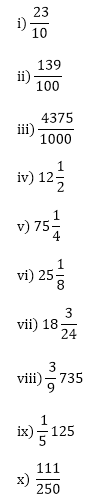×#### Thank you for registering.

One of our academic counsellors will contact you within 1 working day.

Click to Chat

1800-1023-196

+91-120-4616500

CART 0

• 0

MY CART (5)

Use Coupon: CART20 and get 20% off on all online Study Material

ITEM
DETAILS
MRP
DISCOUNT
FINAL PRICE
Total Price: Rs.

There are no items in this cart.
Continue Shopping• Complete JEE Main/Advanced Course and Test Series
• OFFERED PRICE: Rs. 15,900
• View Details

```Chapter 7: Decimals – Exercise 7.4

Question: 1

Express the following fractions as decimals:Solution:

i) 2310 = 20 + 310 = 2010 + 310 = 2 + 310 = 2.3
ii) 139100 = 10 + 30 + 9100 = 100100 + 30100 + 9100 = 1 + 310 + 9100 = 1.39
iii) 43751000 = 4000 + 300 + 70 + 51000 = 40001000 + 3001000 + 701000 + 51000 = 4 + 310 + 7100 + 51000 = 4.375
iv) 1212 = 12 + 12 = 12 + 1(52)(5) = 12 + 510 = 12.5
v) 7514 = 75 + 14 = 75 + 1(254)(25) = 75 + 25100 = 75.25
vi) 2518 = 25+1(1258)(125) = 25 + 1251000 = 25.125
vii) 18324 = 18+324 = 18 + 18 =18 + 125(1125)(8) = 18 +1251000 = 18.125
viii) 39735 = 39 +735 = 39 +15 = 39 + 1(25) (2) = 39 + 210 = 39.2
ix) 15125 =15 + 125 = 15 + 1(1)(425)(4) = 15 + 4100 = 15.04
x)111250 = 111(4250)(4) = 4441000 = 0.444

Question: 2

Express the following decimals as fractions in the lowest form:

i) 0.5
ii) 2.5
iii) 0.60
iv) 0.18
v) 5.25
vi) 7.125
vii) 15.004
viii) 20.375
ix) 600.75

Solution:```### Course Features

• 728 Video Lectures
• Revision Notes
• Previous Year Papers
• Mind Map
• Study Planner
• NCERT Solutions
• Discussion Forum
• Test paper with Video Solution